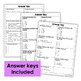Decimals {Practice Pages}Subject
Resource Type
Product Rating
File Type

PDF (Acrobat) Document File

401 KB|28 pages
Share
Product Description
This decimal practice resource includes 25 half page (2 to a page) practice pages for decimals.

These printables would work well for homework, independent practice, classwork, exit slips, and many other uses.

This resource reviews these decimal skills:
Decimal Models
Naming Decimals
Writing Decimals in Word Form
Writing Decimals in Standard Form
Writing Decimals in Expanded Form
Rounding Decimals
Comparing Decimals
Comparing Decimals with Models
Subtracting Decimals with Models
Subtracting Decimals
Decimal Word Problems
Multiplying Decimals and Whole Numbers
Multiplying Decimals and Whole Numbers with Models
Multiplying Decimals
Multiplying Decimals with Models
Multiplying Decimals Word Problems
Dividing Whole Numbers by Decimals
Dividing Decimals by Whole Numbers
Dividing Decimals
Dividing with Models
Dividing Decimals Word Problems
Decimal Word Problems

You may also like:
Complete Guide to Decimals
Decimal of the Day Printables
Decimal Spinner Math Centers
Total Pages
28 pages